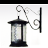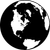# Python Toolbox-populate drop-down with field values

8472
4
01-03-2016 08:36 PMOccasional Contributor

Hi,

There are a few discussions on this topic but I'm not finding the solution I need or it is right in front of my face and I'm missing it completely.

I'm using Desktop 10.3.   I'm am trying to create a geoprocessing tool with the input dialogue box requesting the user to select:

1. Feature class  e.g US States

2. Field with the States name in the Feature Class  e.g. stateNames

3. A drop-down of the 50 states where the user can select one of the states.

I'm trying to use the python toolbox tool template (portion shown below).  I'm able to populate a drop-down of the fields and now need to have the user select a value from the field.

Any suggestions?

Thanks

Brian

class tool(object):

def __init__(self):

"""Define the tool (tool name is the name of the class)."""

self.label = "tool"

self.description = ""

self.canRunInBackground = True

def getParameterInfo(self):

# first paramter

in_feature = arcpy.Parameter(

displayName = "Feature with States",

name = "in_feature",

datatype = "Feature Layer",

parameterType = "Required",

direction = "Input")

stateField = arcpy.Parameter(

displayName = "Field with State Names",

name = "stateField",

datatype = "Field",

parameterType = "Required",

direction = "Input")

stateField.filter.list = ['Text']

stateField.parameterDependencies = [in_feature.name]

stateName = arcpy.Parameter(

displayName = "Select one State",

name = "stateName",

datatype = "GPValueTable",

parameterType = "Required",

direction = "Input")

return

def updateParameters(self, parameters):

"""Modify the values and properties of parameters before internal

validation is performed.  This method is called whenever a parameter

has been changed."""

return

1 Solution

Accepted SolutionsbyMVP Regular Contributor

I thought there was a way to do this easily with parameterDependencies, but can't get that to work. But below is another way:

```class Tool(object):
def __init__(self):
"""Define the tool (tool name is the name of the class)."""
self.label = "Tool"
self.description = ""
self.canRunInBackground = False

def getParameterInfo(self):
"""Define parameter definitions"""

in_feature = arcpy.Parameter(
displayName = "Feature with States",
name = "in_feature",
datatype = "Feature Layer",
parameterType = "Required",
direction = "Input")

stateField = arcpy.Parameter(
displayName = "Field with State Names",
name = "stateField",
datatype = "Field",
parameterType = "Required",
direction = "Input")

stateField.parameterDependencies = [in_feature.name]

stateName = arcpy.Parameter(
displayName = "Select one State",
name = "stateName",
datatype = "GPString",
parameterType = "Required",
direction = "Input")

stateName.filter.type = "ValueList"
stateName.filter.list = []

return [in_feature, stateField, stateName]

def updateParameters(self, parameters):
"""Modify the values and properties of parameters before internal
validation is performed.  This method is called whenever a parameter
has been changed."""
if parameters.value:
with arcpy.da.SearchCursor(parameters.valueAsText, parameters.valueAsText) as rows:
parameters.filter.list = sorted(list(set([row for row in rows])))
else:
parameters.filter.list = []

return```
4 RepliesbyMVP Regular Contributor

I thought there was a way to do this easily with parameterDependencies, but can't get that to work. But below is another way:

```class Tool(object):
def __init__(self):
"""Define the tool (tool name is the name of the class)."""
self.label = "Tool"
self.description = ""
self.canRunInBackground = False

def getParameterInfo(self):
"""Define parameter definitions"""

in_feature = arcpy.Parameter(
displayName = "Feature with States",
name = "in_feature",
datatype = "Feature Layer",
parameterType = "Required",
direction = "Input")

stateField = arcpy.Parameter(
displayName = "Field with State Names",
name = "stateField",
datatype = "Field",
parameterType = "Required",
direction = "Input")

stateField.parameterDependencies = [in_feature.name]

stateName = arcpy.Parameter(
displayName = "Select one State",
name = "stateName",
datatype = "GPString",
parameterType = "Required",
direction = "Input")

stateName.filter.type = "ValueList"
stateName.filter.list = []

return [in_feature, stateField, stateName]

def updateParameters(self, parameters):
"""Modify the values and properties of parameters before internal
validation is performed.  This method is called whenever a parameter
has been changed."""
if parameters.value:
with arcpy.da.SearchCursor(parameters.valueAsText, parameters.valueAsText) as rows:
parameters.filter.list = sorted(list(set([row for row in rows])))
else:
parameters.filter.list = []

return```Occasional Contributor

Luke,  Thank you so very much. It works great.  Brianby
Occasional Contributor

Thanks for this!Frequent Contributor

I am new to this....how is the class called from Python and used?

Is there a simply example of this code in its entirety where when you run it you just see the dropdown and the populated list?